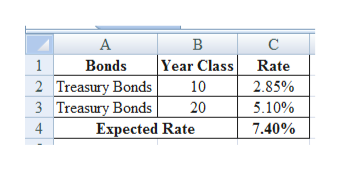The Wall Street Journal reports that the current rate on 10-year Treasury bonds is 2.85 percent and the rate on 20-year Treasury bonds is 5.10 percent. Assume that the maturity risk premium is zero. Calculate the expected rate on a 10-year Treasury bond purchased ten years from today, E(10r10). (Do not round intermediate calculations. Round your answer to 2 decimal places.)Expected rate  ____.__%

Question

The Wall Street Journal reports that the current rate on 10-year Treasury bonds is 2.85 percent and the rate on 20-year Treasury bonds is 5.10 percent. Assume that the maturity risk premium is zero. Calculate the expected rate on a 10-year Treasury bond purchased ten years from today, E(10r10). (Do not round intermediate calculations. Round your answer to 2 decimal places.)

Expected rate  ____.__%

Step 1

Calculation of Expected Rate:

The expected rate of 10-year Treasury Bond is 7.40%.

...help_outlineImage TranscriptioncloseВ |Year Class Rate Bonds 2 Treasury Bonds 3 Treasury Bonds Expected Rate 1 10 2.85% 5.10% 20 4 7.40% fullscreen

Want to see the full answer?

See Solution

Want to see this answer and more?

Our solutions are written by experts, many with advanced degrees, and available 24/7

See Solution
Tagged in